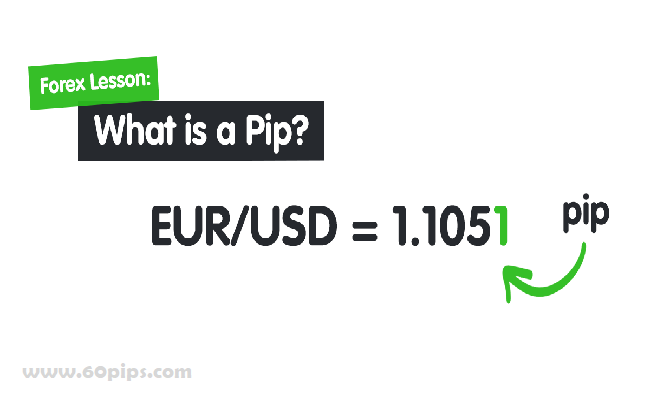### What is PIP?

Pip is an acronym for “percentage in point” or “price interest point.” A pip is the smallest price move that an exchange rate can make based on forex market convention. Most currency pairs are priced out to four decimal places and the pip change is the last (fourth) decimal point. A pip is thus equivalent to 1/100 of 1% or one basis point.

### How Pips Work

A pip is a basic concept of foreign exchange (forex). Forex pairs are used to disseminate exchange quotes through bid and ask quotes that are accurate to four decimal places. In simpler terms, forex traders buy or sell a currency whose value is expressed in relationship to another currency.

Movement in the exchange rate is measured by pips. Since most currency pairs are quoted to a maximum of four decimal places, the smallest change for these pairs is 1 pip. The value of a pip can be calculated by dividing 1/10,000 or 0.0001 by the exchange rate.

### Pips and Profitability

The movement of a currency pair determines whether a trader made a profit or loss from the positions at the end of the day. A trader who buys the EUR/USD will profit if the euro increases in value relative to the US dollar. If the trader bought the euro for 1.1835 and exited the trade at 1.1901, they would make 1.1901 – 1.1835 = 66 pips on the trade.

##### Now let’s consider a trader who buys the Japanese Yen by selling USD/JPY at 112.06. The trader loses 3 pips on the trade if closed at 112.09 but profits by 5 pips if the position is closed at 112.01.

While the difference looks small in the multi-trillion dollar foreign exchange market, gains and losses can add up quickly. For example, if a \$10 million position in this set-up is closed at 112.01, the trader will book a \$10 million x (112.06 – 112.01) = \$500,000 profit. This profit in US dollars is calculated as \$500,000/112.01 = \$4,463.89.